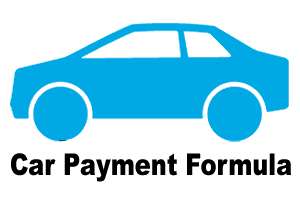# How To Calculate Monthly Car Payment?

If you are planning to buy a car and want to get a good deal you should do some homework, you need to learn how to calculate car payment ahead of time. You can calculate your monthly car payments or estimate the total cost of your car purchase by following the car payment formula.For example, you borrowed RM40,000 for your new Honda City for 5 years, with an interest rate of 2.9%. Then, you are expected to pay RM763.33 monthly for the next 5 years (60 months).

Steps On How To Calculate Your Monthly Payments

Car loan principal = RM 75,000 (Assume)
Down payment = RM 35,000 (Assume)
Interest rate 2.9% = RM 40,000 x 2.9/100
= RM 1160/year

Loan paid for 5 years tenure (Assume) = Interest paid + Loan amount
= (RM 1160 x 5) + RM 40,000
= RM 45,800

Monthly car payment = RM 45,800/5 x 12
= RM 763.33

In conclusion, car loan payment calculation require basic information, such as the loan amount, interest rate and the duration of the loan in order to calculate the monthly car payments. So, based on the above calculation and formula, monthly car payments can be calculated manually using a calculator.

## 2 thoughts on “How To Calculate Monthly Car Payment?”

1.Ian Kree says:

Thank you or sharing. Most Malaysian do not know how to calculate car loan (and other loans) – resulting in unwise financial decisions. “Calculate and think before you spend and invest.”

2.Calvyn says:

this is a very clear calculation… now I know how my interest rate be count